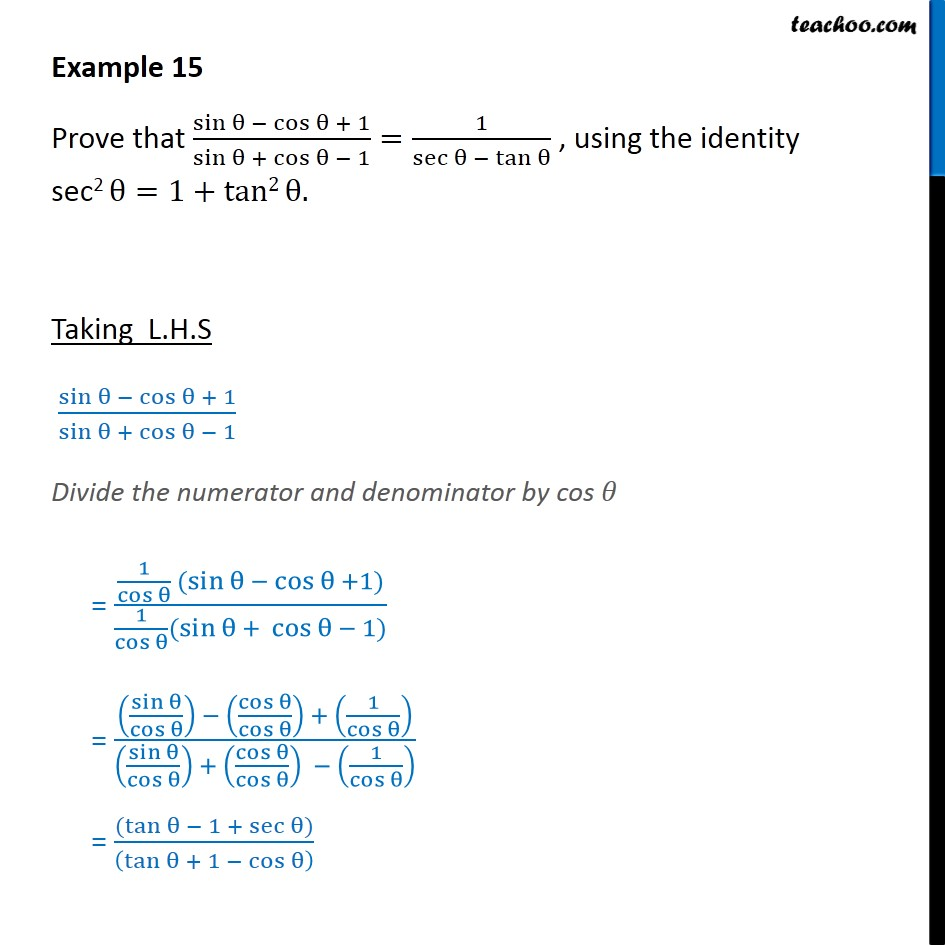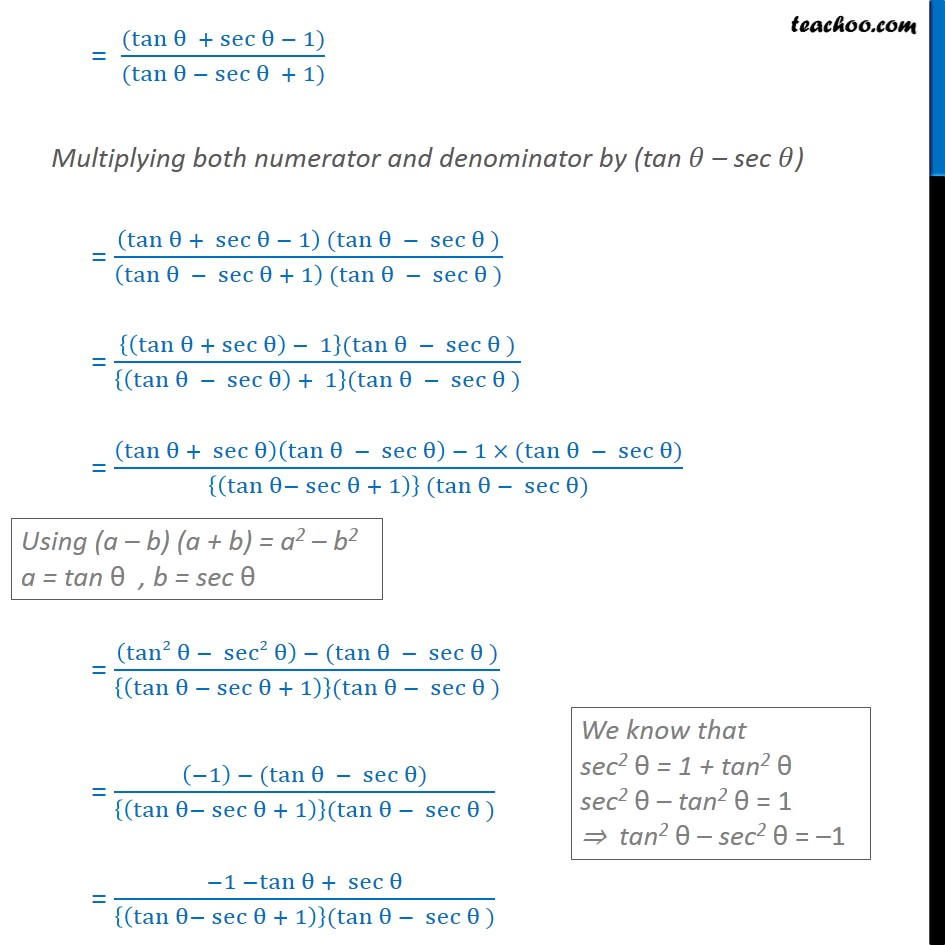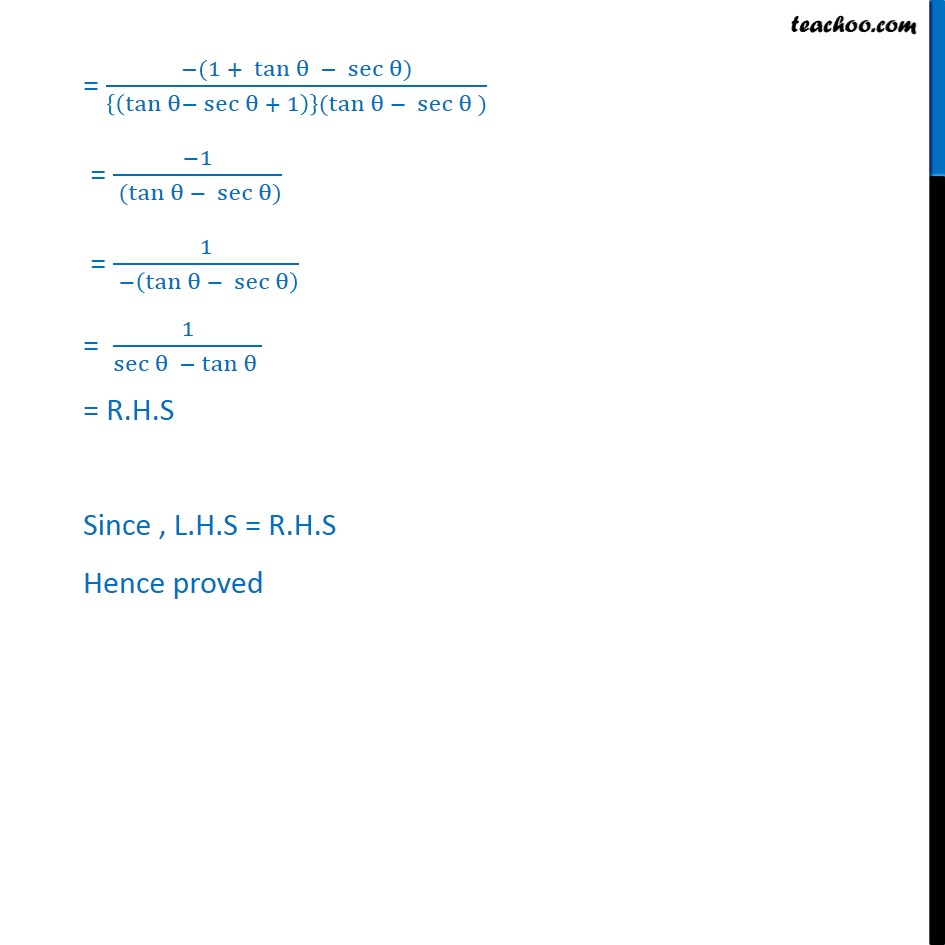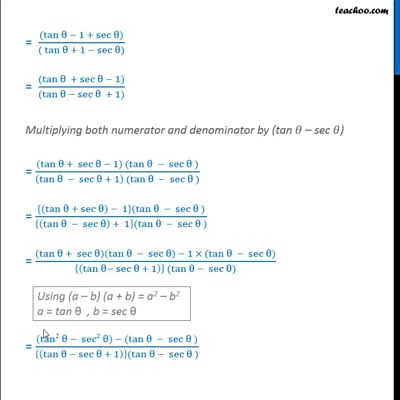Examples

Chapter 8 Class 10 Introduction to Trignometry
Serial order wiseThis video is only available for Teachoo black users

Introducing your new favourite teacher - Teachoo Black, at only ₹83 per month

### Transcript

Example 15 Prove that (sin θ − cos θ + 1)/(sin θ + cos θ − 1)=1/(sec θ − tan θ) , using the identity sec2 θ=1+tan2 θ. Taking L.H.S (sin⁡θ − cos θ + 1)/(sin θ + cos θ − 1) Divide the numerator and denominator by cos 𝜃 = (1/(cos θ) (sin θ − cos θ +1))/(1/(cos θ)(sin θ + cos θ − 1)) = (((sin θ)/(cos θ)) − ((cos θ)/(cos θ)) + (1/(cos θ)))/(((sin θ)/(cos θ)) + ((cos θ)/(cos θ)) − (1/(cos θ)) ) = ((tan θ − 1 + sec θ))/((tan θ + 1 − cos θ) ) = ((tan θ + sec θ − 1))/((tan θ − sec θ + 1)) Multiplying both numerator and denominator by (tan 𝜃 – sec 𝜃) = ((tan θ + sec θ − 1) (tan θ − sec θ ))/((tan θ − sec θ + 1) (tan θ − sec θ )) = ({(tan⁡θ + sec⁡θ ) − 1}(tan θ − sec θ ))/({(tan θ − sec θ) + 1}(tan θ − sec θ )) = ((tan θ + sec θ)(tan θ − sec θ) − 1 × (tan θ − sec θ))/({(tan⁡θ− sec⁡θ + 1)} (tan θ − sec θ)) = ((tan2 θ − sec2 θ) − (tan θ − sec θ ))/({(tan⁡θ − sec⁡θ + 1)}(tan θ − sec θ )) = ((−1) − (tan θ − sec θ))/({(tan⁡θ− sec⁡θ + 1)}(tan θ − sec θ )) = (−1 −tan θ + sec θ)/({(tan⁡θ− sec⁡θ + 1)}(tan θ − sec θ )) = (−(1 + tan θ − sec θ))/({(tan⁡θ− sec⁡θ + 1)}(tan θ − sec θ )) = (−1)/( (tan θ − sec θ)) = 1/( −(tan θ − sec θ) ) = 1/(sec θ − tan θ ) = R.H.S Since , L.H.S = R.H.S Hence proved